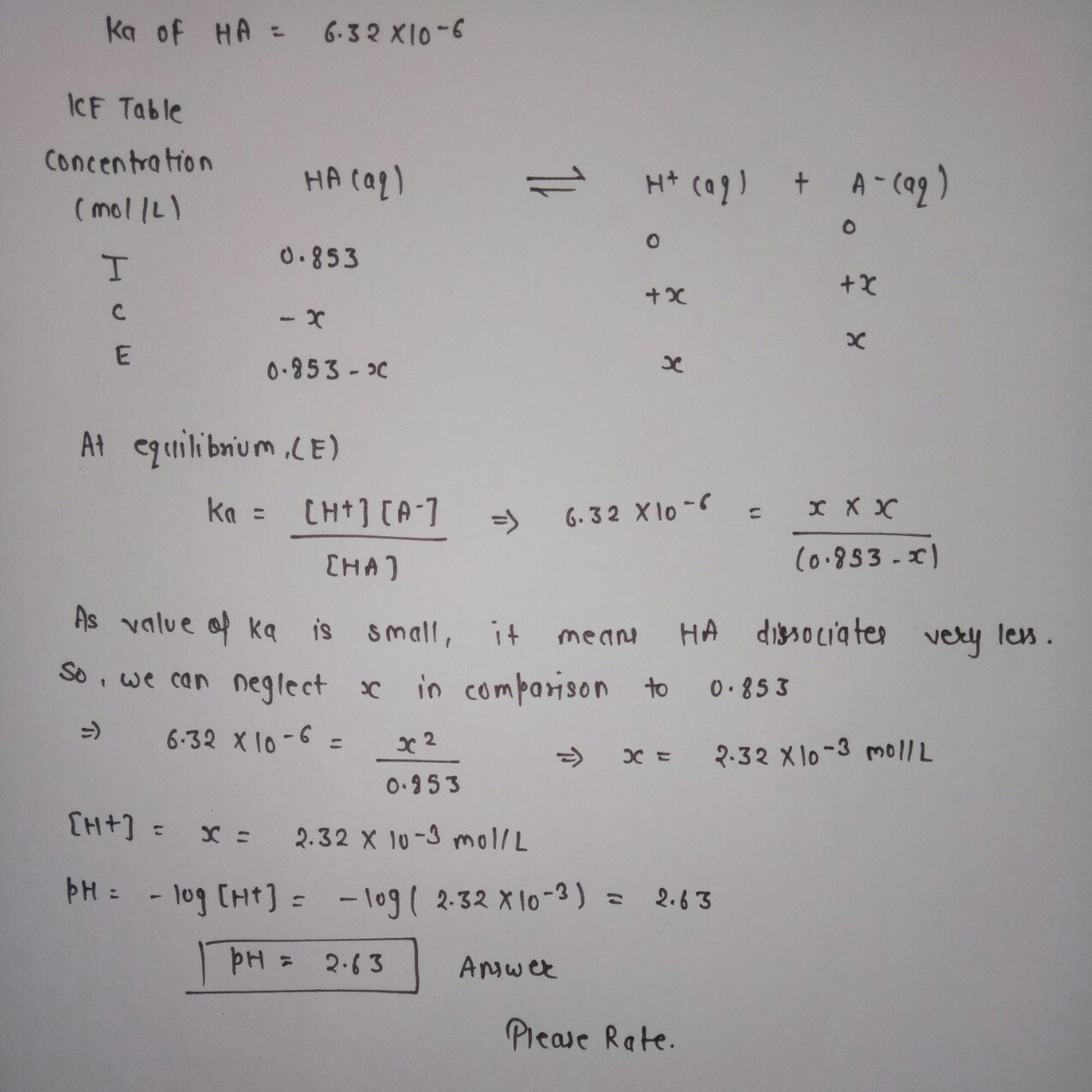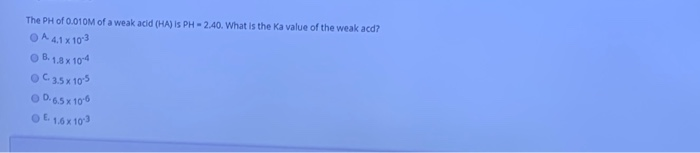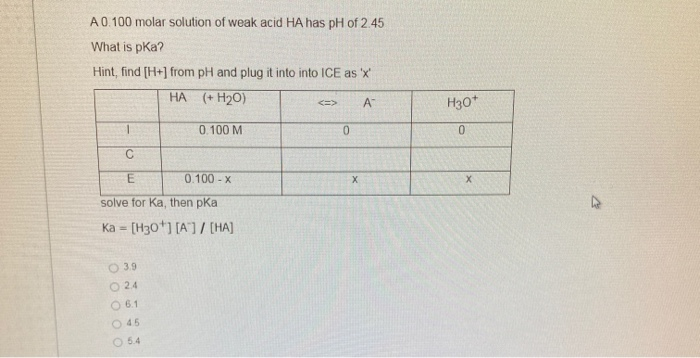Question

Consider a weak acid "HA". What is the pH of a 0.853 mol L-1 solution of HA? The KA of HA is 6.32 x 10-6#### Earn Coins

Coins can be redeemed for fabulous gifts.

Similar Homework Help Questions
• ### What is the pH of a buffer prepared by adding 0.506 mol of the weak acid HA to 0.305 mol of NaA in 2.00  L of solu...

What is the pH of a buffer prepared by adding 0.506 mol of the weak acid HA to 0.305 mol of NaA in 2.00  L of solution? The dissociation constant Ka of HA is 5.66*10^-7. pH = __________ What is the \rm pH after 0.150 mol of \rm HCl is added to the buffer from Part A? Assume no volumechange on the addition of the acid. pH = _____________ What is the \rm pH after 0.195 mol of \rm NaOH...

• ### Question 1    :   HA is a weak acid. Its ionization constant, Ka, is 1.2 x 10-13....

Question 1    :   HA is a weak acid. Its ionization constant, Ka, is 1.2 x 10-13. Calculate the pH of an aqueous solution with an initial NaA concentration of 0.075 M.   Question 2    :   We place 0.143 mol of a weak acid, HA, in enough water to produce 1.00 L of solution. The final pH of the solution is 1.28 . Calculate the ionization contant, Ka, of HA.   Question 3    :   We place 0.661 mol of a weak acid, HA,...

• ### What is the pH of a 0.030 M solution of weak acid HA for which Ka...

What is the pH of a 0.030 M solution of weak acid HA for which Ka = 6.6 x 10-5?

• ### Part A What is the pH of a buffer prepared by adding 0.506 mol of the weak acid HA to 0.608 mol of NaA in 2.00 L of so...

Part A What is the pH of a buffer prepared by adding 0.506 mol of the weak acid HA to 0.608 mol of NaA in 2.00 L of solution? The dissociation constant Ka of HA is 5.66×10−7. Express the pH numerically to three decimal places. pH = SubmitHintsMy AnswersGive UpReview Part Part B What is the pH after 0.150 mol of HCl is added to the buffer from Part A? Assume no volume change on the addition of the acid....

• ### We place 0.134 mol of a weak acid, HA, in enough water to produce 1.00 L...

We place 0.134 mol of a weak acid, HA, in enough water to produce 1.00 L of solution. The final pH of the solution is 1.18 . Calculate the ionization contant, Ka, of HA

• ### Please answer all the questions #1. HA is a weak acid. an aqueous solution of HA...

Please answer all the questions #1. HA is a weak acid. an aqueous solution of HA was prepared by dissolving 0.185 mol of HA and diluted to 525mL. The pH of solution was 3.93 at 25.0°C. The Ka of HA is _____. #2. What is the pH of a solution at 25°C with[H^+] = 2.50 • 10^-5?

• ### The PH of 0.010M of a weak acid (HA) is PH - 2.40. What is the...The PH of 0.010M of a weak acid (HA) is PH - 2.40. What is the Ka value of the weak acd? O A 41 x 10²3 B. 1.8 x 10-4 0C 3.5x10-5 0.65 x 10-6 E. 1.6x109

• ### 1) A 0.224 M solution of a weak acid (HA) is made. The Ka for this acid is 7.73 ⋅ 10-5 . -What is the pH of this solutio...

1) A 0.224 M solution of a weak acid (HA) is made. The Ka for this acid is 7.73 ⋅ 10-5 . -What is the pH of this solution? -What is the pOH of this solution? 2)A 0.238 M solution of a weak base (B:) is made. The Kb for this acid is 7.34⋅10-3. -What is the pOH of this solution? -What is the pH of this solution?

• ### The pH of a 0.00156 M solution of a certain weak acid, HA is found to...

The pH of a 0.00156 M solution of a certain weak acid, HA is found to be 3.64. What is the Ka of acid? 2.37 x 10-4 3.94 x 10-5 2.81 x 102 4.29 x 10-4

• ### A 0.100 molar solution of weak acid HA has pH of 2.45 What is pka? Hint,...A 0.100 molar solution of weak acid HA has pH of 2.45 What is pka? Hint, find [H+] from pH and plug it into into ICE as 'X' HA (+H20) А" <> H30* 0.100 M 0 0 С E 0.100 - X х х solve for Ka, then pka Ka = [H30*1 [A]/[HA] 39 24 6.1 45 5.4 Consider the titration of 25.00 ml of 0.100 MHA with 25.0 0.100 M NaOH. HA +H20 --> A™ + H307 The Ka...